# Welcome to Electrostatics Outline 1 Coulombs Law 2

• Slides: 84Welcome… …to Electrostatics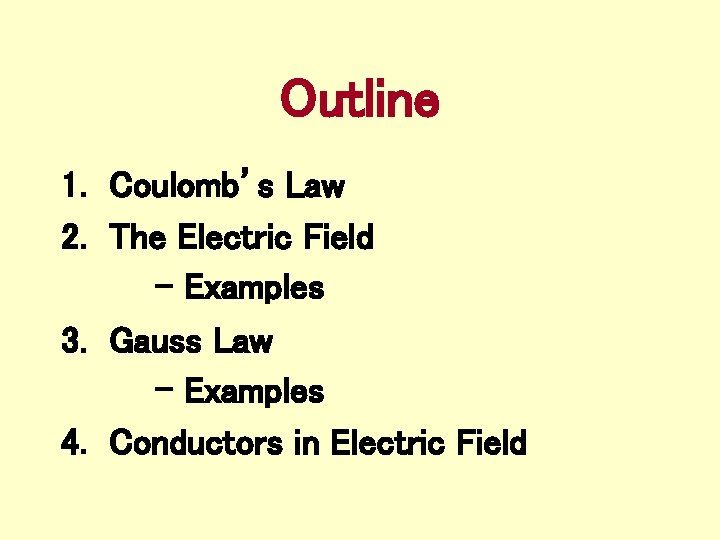Outline 1. Coulomb’s Law 2. The Electric Field - Examples 3. Gauss Law - Examples 4. Conductors in Electric FieldCoulomb’s Law Coulomb’s law quantifies the magnitude of the electrostatic force. Coulomb’s law gives the force (in Newtons) between charges q 1 and q 2, where r 12 is the distance in meters between the charges, and k=9 x 109 N·m 2/C 2.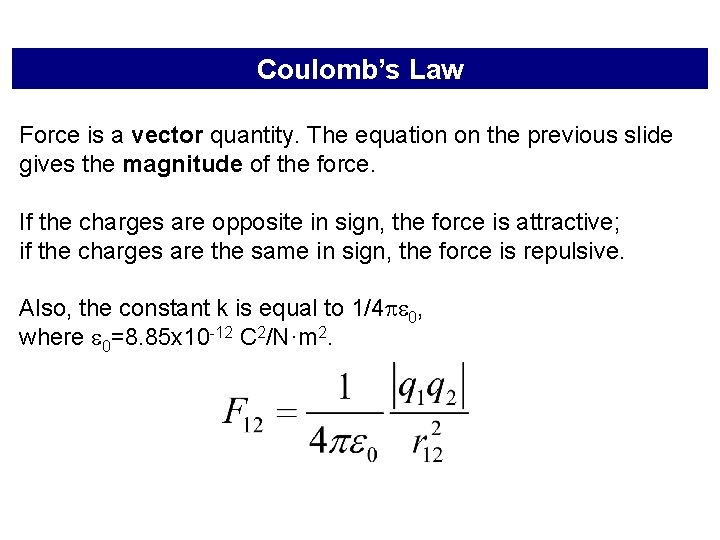Coulomb’s Law Force is a vector quantity. The equation on the previous slide gives the magnitude of the force. If the charges are opposite in sign, the force is attractive; if the charges are the same in sign, the force is repulsive. Also, the constant k is equal to 1/4 0, where 0=8. 85 x 10 -12 C 2/N·m 2.Coulomb’s Law • If q 1 and q 2 are located at points having position vectors r 1 and r 2 then the Force F 12 is given by;The equation is valid for point charges. If the charged objects are spherical and the charge is uniformly distributed, r 12 is the distance between the centers of the spheres. r 12 - + If more than one charge is involved, the net force is the vector sum of all forces (superposition). For objects with complex shapes, you must add up all the forces acting on each separate charge (turns into calculus!). + + + - - -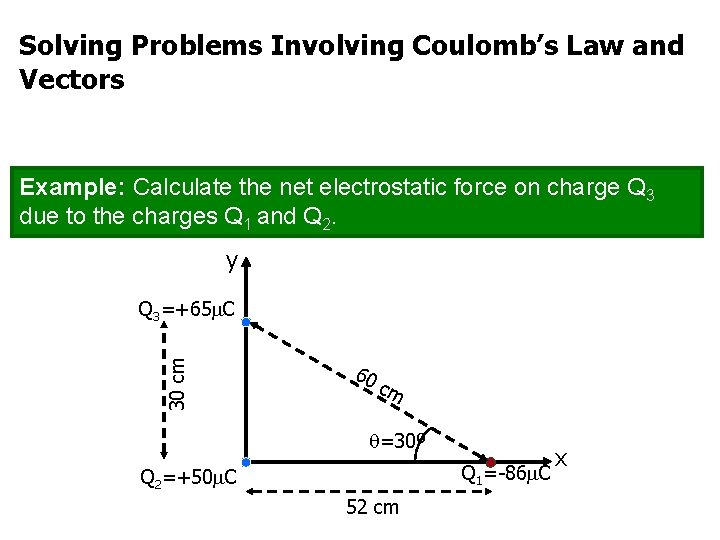Solving Problems Involving Coulomb’s Law and Vectors Example: Calculate the net electrostatic force on charge Q 3 due to the charges Q 1 and Q 2. y 30 cm Q 3=+65 C 60 cm =30º Q 1=-86 C Q 2=+50 C 52 cm xStep 0: Think! This is a Coulomb’s Law problem (all we have to work with, so far). We only want the forces on Q 3. Forces are additive, so we can calculate F 32 and F 31 and add the two. If we do our vector addition using components, we must resolve our forces into their x- and y-components.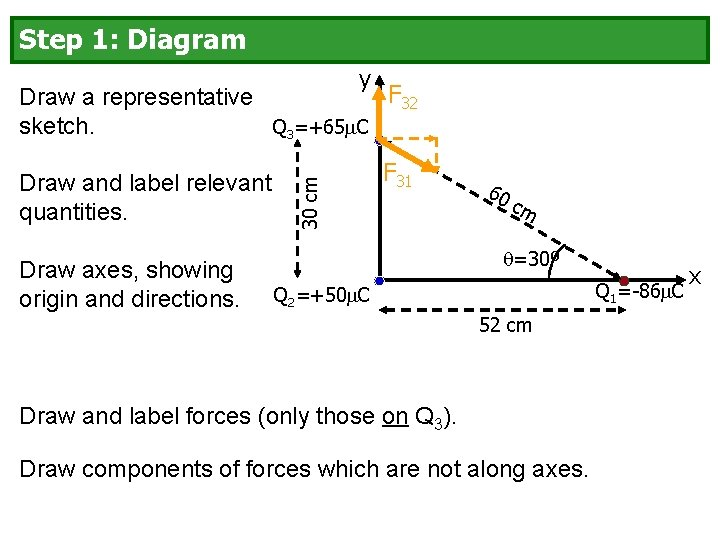Step 1: Diagram y Draw and label relevant quantities. Draw axes, showing origin and directions. 30 cm F 32 Draw a representative sketch. Q 3=+65 C F 31 60 cm =30º Q 1=-86 C Q 2=+50 C 52 cm Draw and label forces (only those on Q 3). Draw components of forces which are not along axes. xStep 2: Starting Equation y F 32 30 cm Q 3=+65 C F 31 60 cm =30º Q 1=-86 C Q 2=+50 C 52 cm “Do I have to put in the absolute value signs? ” xStep 3: Replace Generic Quantities by Specifics y F 32 r 32=30 cm Q 3=+65 C F 31 r 3 1 =6 0 c =30º Q 1=-86 C Q 2=+50 C 52 cm (from diagram) F 32, y = 330 N and F 32, x = 0 N. m xStep 3 (continued) y F 32 r 32=30 cm Q 3=+65 C (+ sign comes from diagram) F 31 r 3 1 =6 0 c m =30º Q 1=-86 C Q 2=+50 C 52 cm (- sign comes from diagram) You would get F 31, x = +120 N and F 31, y = -70 N. x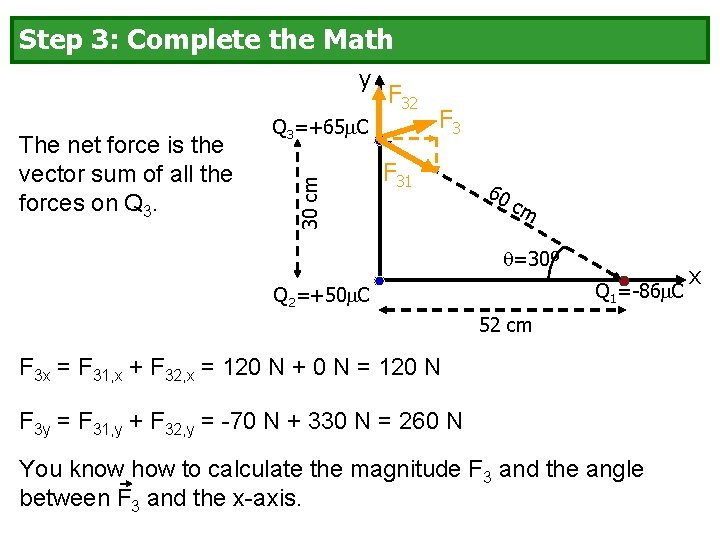Step 3: Complete the Math y Q 3=+65 C 30 cm The net force is the vector sum of all the forces on Q 3. F 32 F 31 60 cm =30º Q 1=-86 C Q 2=+50 C 52 cm F 3 x = F 31, x + F 32, x = 120 N + 0 N = 120 N F 3 y = F 31, y + F 32, y = -70 N + 330 N = 260 N You know how to calculate the magnitude F 3 and the angle between F 3 and the x-axis. x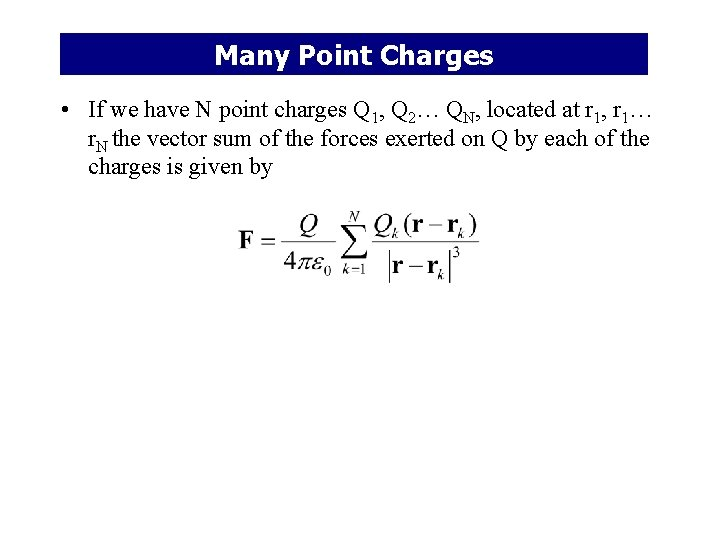Many Point Charges • If we have N point charges Q 1, Q 2… QN, located at r 1, r 1… r. N the vector sum of the forces exerted on Q by each of the charges is given byCoulomb’s Law: The Big Picture Coulomb's Law quantifies the interaction between charged particles. r 12 + - Q 1 Q 2 Coulomb’s Law was discovered through decades of experiment. By itself, it is just “useful. " Is it part of something bigger?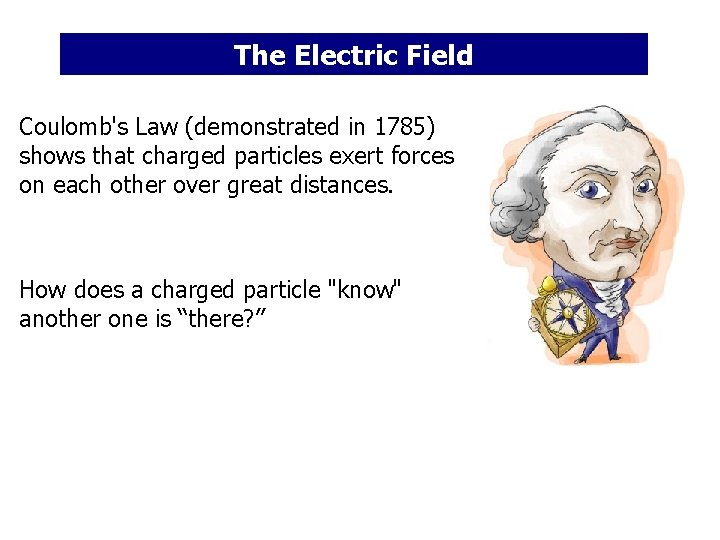The Electric Field Coulomb's Law (demonstrated in 1785) shows that charged particles exert forces on each other over great distances. How does a charged particle "know" another one is “there? ”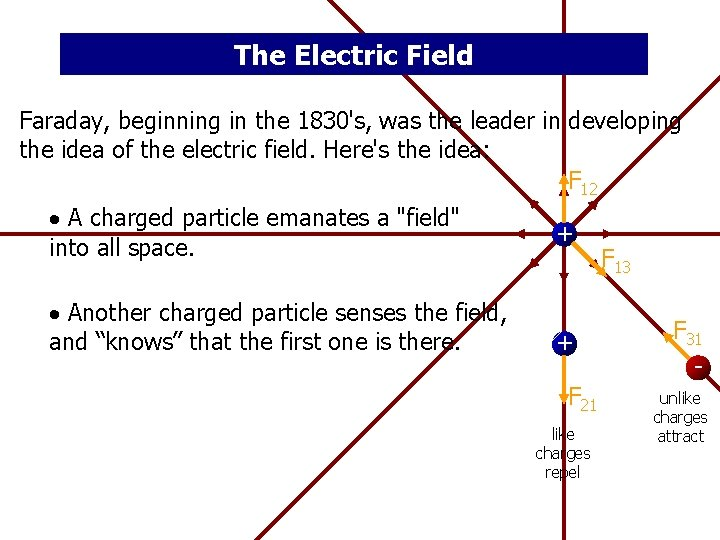The Electric Field Faraday, beginning in the 1830's, was the leader in developing the idea of the electric field. Here's the idea: F 12 A charged particle emanates a "field" into all space. Another charged particle senses the field, and “knows” that the first one is there. + + F 21 like charges repel F 13 F 31 unlike charges attract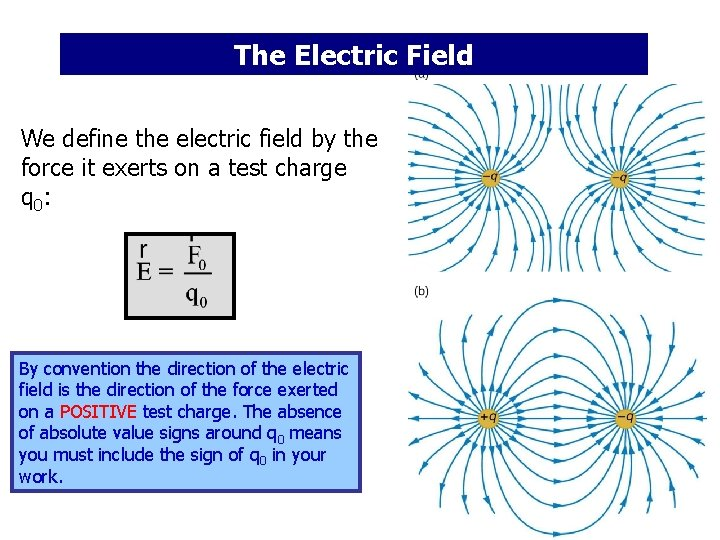The Electric Field We define the electric field by the force it exerts on a test charge q 0: By convention the direction of the electric field is the direction of the force exerted on a POSITIVE test charge. The absence of absolute value signs around q 0 means you must include the sign of q 0 in your work.The Electric Field If the test charge is "too big" it perturbs the electric field, so the “correct” definition is You won’t be required to use this version of the equation. Any time you know the electric field, you can use this equation to calculate the force on a charged particle in that electric field.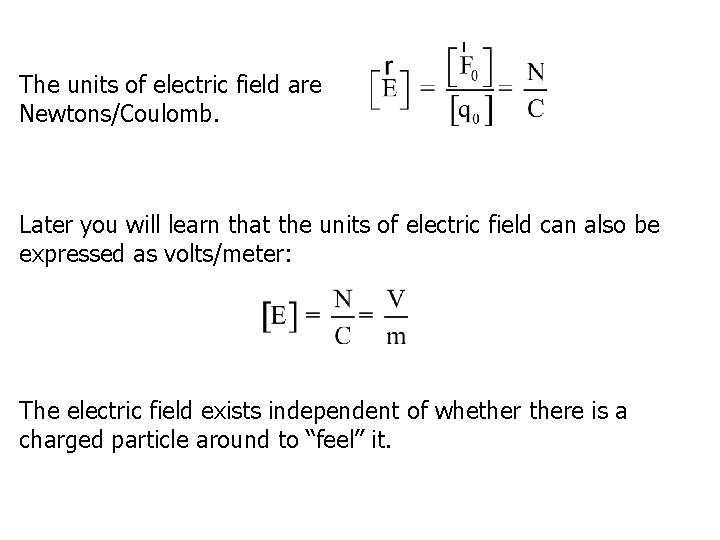The units of electric field are Newtons/Coulomb. Later you will learn that the units of electric field can also be expressed as volts/meter: The electric field exists independent of whethere is a charged particle around to “feel” it.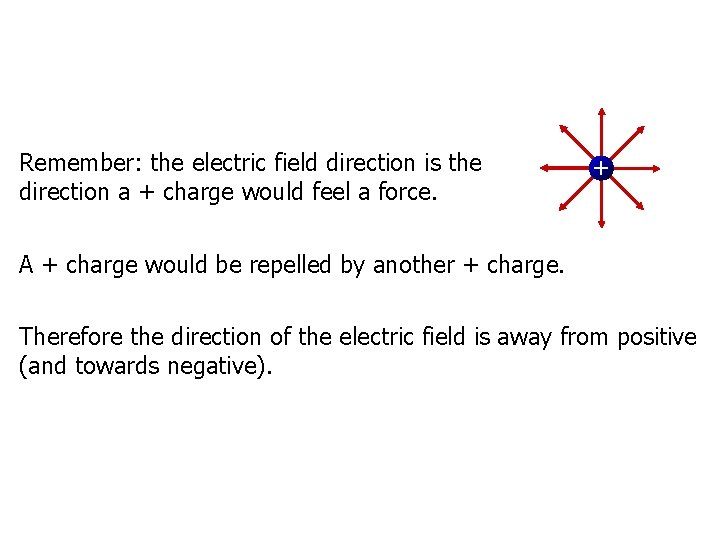Remember: the electric field direction is the direction a + charge would feel a force. + A + charge would be repelled by another + charge. Therefore the direction of the electric field is away from positive (and towards negative).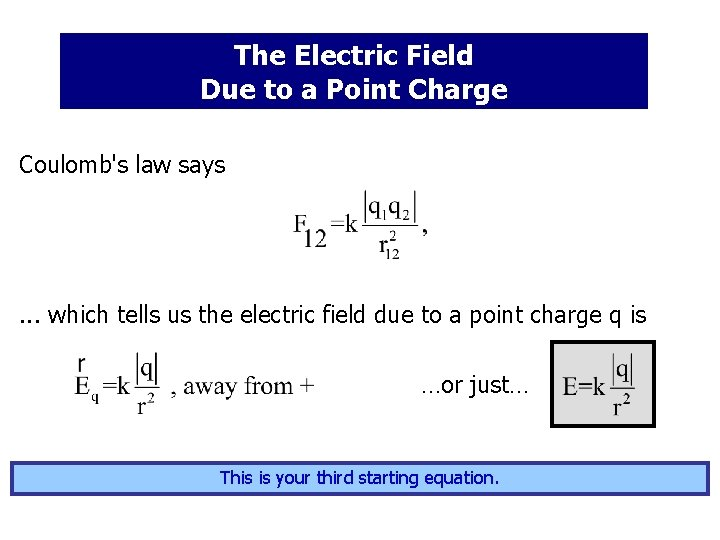The Electric Field Due to a Point Charge Coulomb's law says . . . which tells us the electric field due to a point charge q is …or just… This is your third starting equation.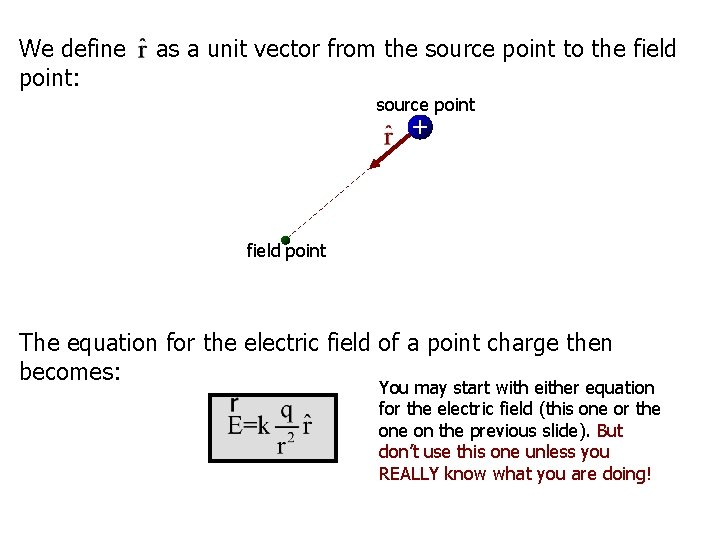We define point: as a unit vector from the source point to the field source point + field point The equation for the electric field of a point charge then becomes: You may start with either equation for the electric field (this one or the on the previous slide). But don’t use this one unless you REALLY know what you are doing!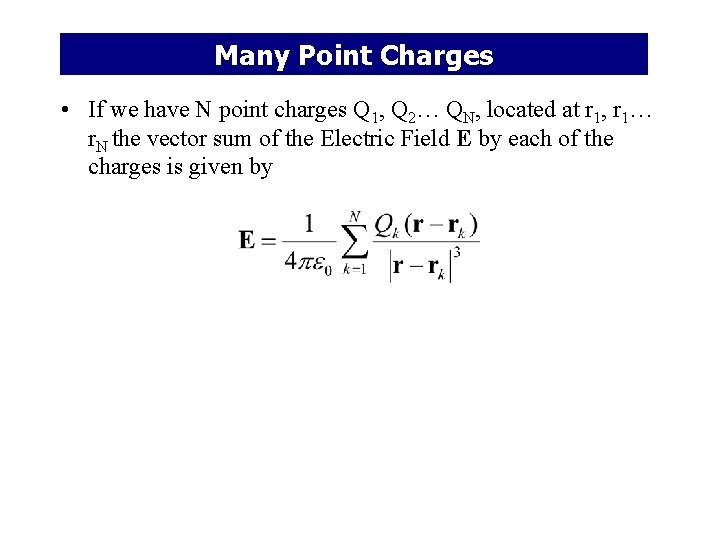Many Point Charges • If we have N point charges Q 1, Q 2… QN, located at r 1, r 1… r. N the vector sum of the Electric Field E by each of the charges is given by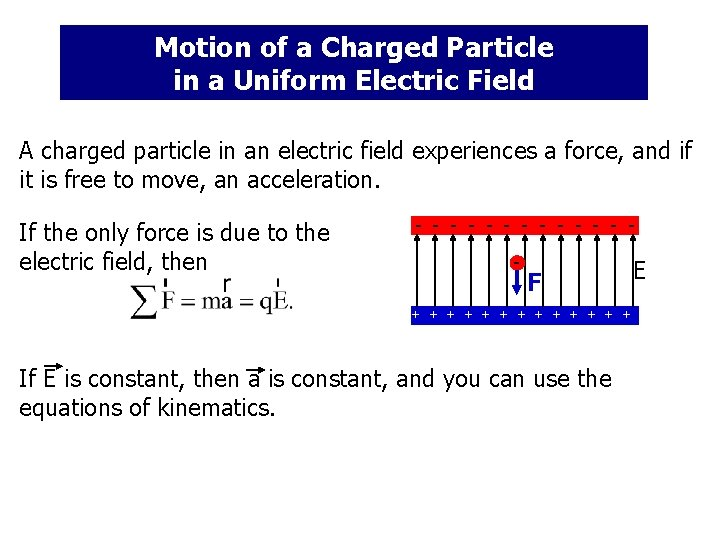Motion of a Charged Particle in a Uniform Electric Field A charged particle in an electric field experiences a force, and if it is free to move, an acceleration. If the only force is due to the electric field, then - - - - F + + + + If E is constant, then a is constant, and you can use the equations of kinematics. E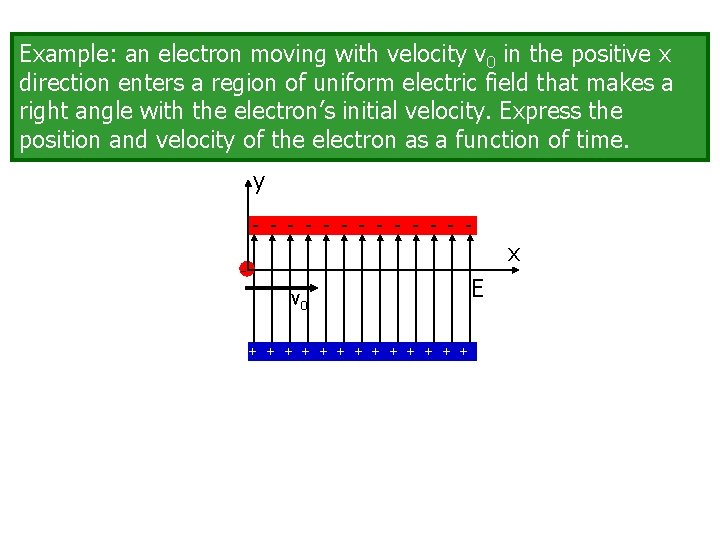Example: an electron moving with velocity v 0 in the positive x direction enters a region of uniform electric field that makes a right angle with the electron’s initial velocity. Express the position and velocity of the electron as a function of time. y - - - - x - v 0 + + + + EThe Electric Field Due to a Collection of Point Charges The electric field due to a small "chunk" q of charge is unit vector from q to wherever you want to calculate E The electric field due to collection of "chunks" of charge is As q dq 0, the sum becomes an integral.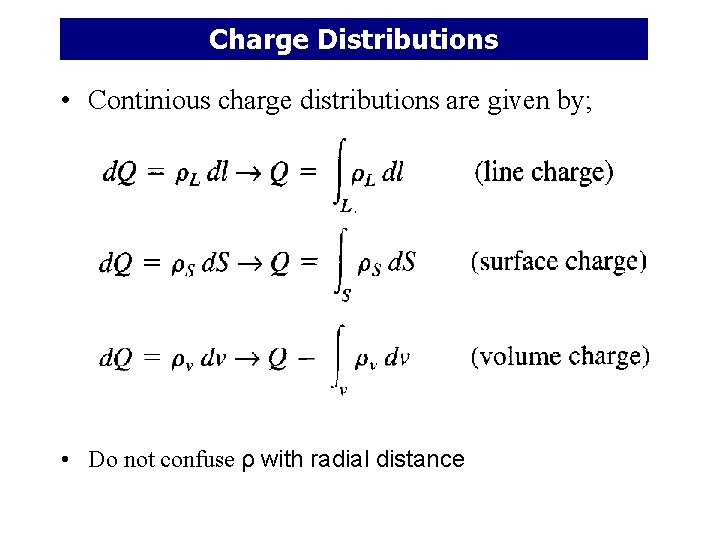Charge Distributions • Continious charge distributions are given by; • Do not confuse ρ with radial distance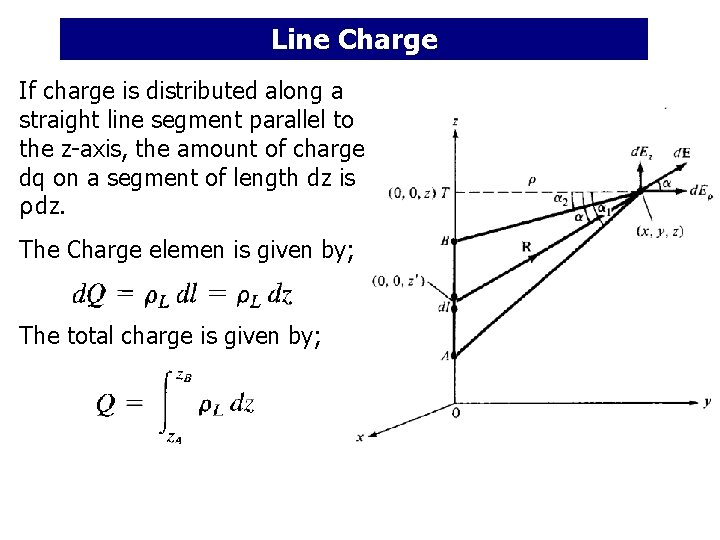Line Charge If charge is distributed along a straight line segment parallel to the z-axis, the amount of charge dq on a segment of length dz is ρ dz. The Charge elemen is given by; The total charge is given by;Line Charge The electric field at point P due to the charge dq is The field point is denoted by (x, y, z) while the source point is denoted by (x’, y’, z’) I’m assuming positively charged objects in these “distribution of charges” slides.Line Charge Definitions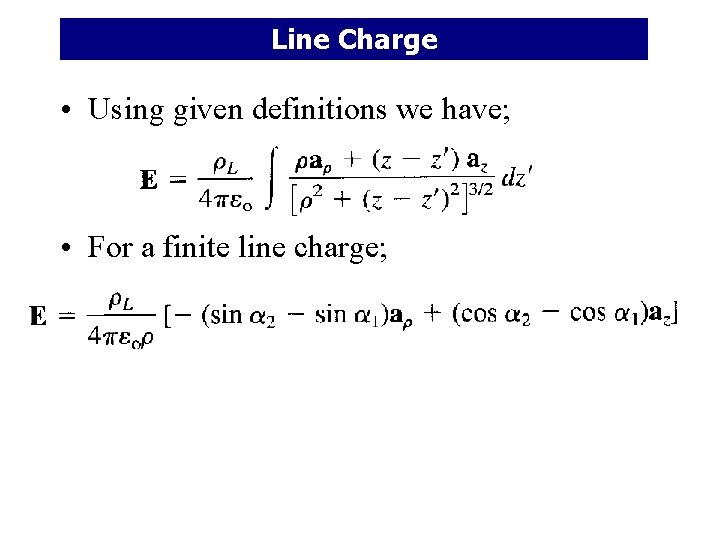Line Charge • Using given definitions we have; • For a finite line charge;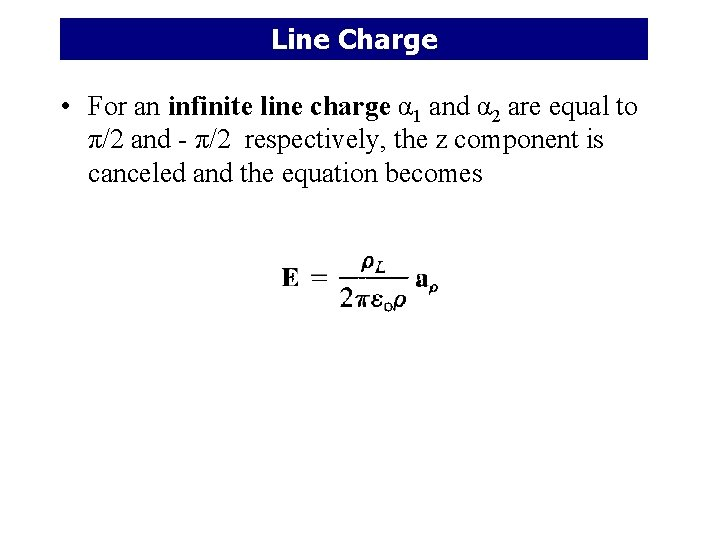Line Charge • For an infinite line charge α 1 and α 2 are equal to π/2 and - π/2 respectively, the z component is canceled and the equation becomes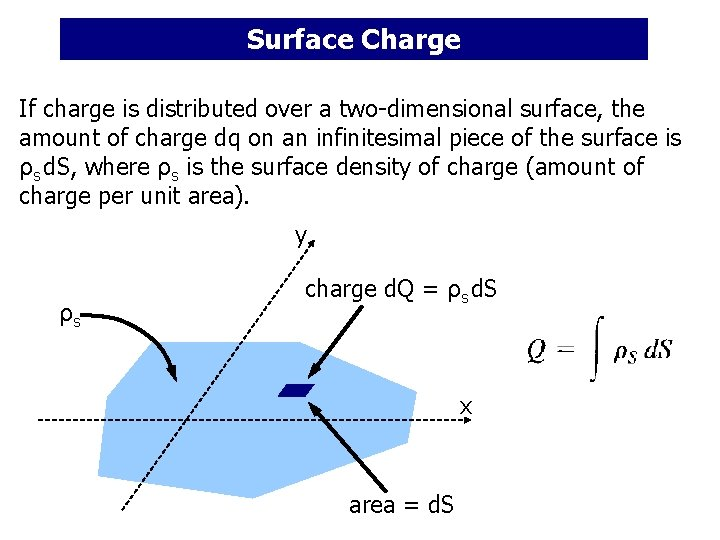Surface Charge If charge is distributed over a two-dimensional surface, the amount of charge dq on an infinitesimal piece of the surface is ρs d. S, where ρs is the surface density of charge (amount of charge per unit area). y ρs charge d. Q = ρs d. S x area = d. SSurface Charge y P d. E r’ x The electric field at P due to the charge dq isSurface Charge P d. E r’ x The net electric field at P due to the entire surface of charge isSurface Charge • Definitions • And the E field becomes;Surface Charge • After evaluating the previous integration; • Or a more general formula is an is a unit vector normal to the sheet • Note that the Electric Field is independent of the distance between the sheet and the point of observation PVolume Charge The net electric field at P due to a three-dimensional distribution of charge is… z E P r’ x yVolume Charge • For a sphere with radius a centered at the origin • The E field due to elementary volume charge is given by;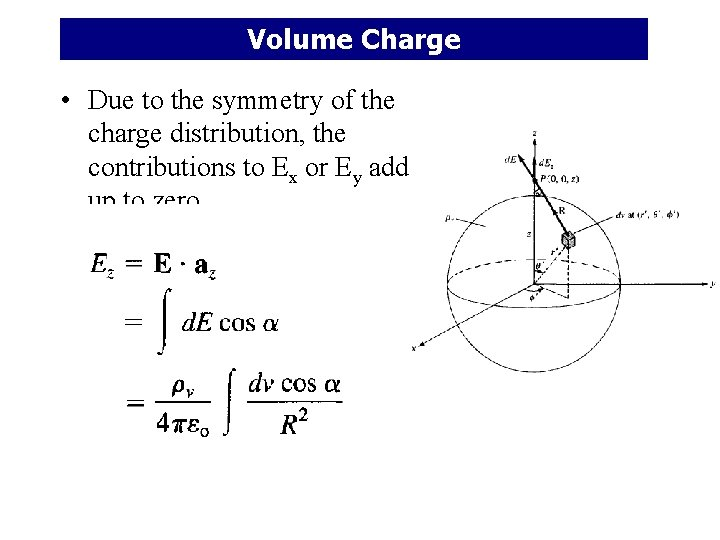Volume Charge • Due to the symmetry of the charge distribution, the contributions to Ex or Ey add up to zero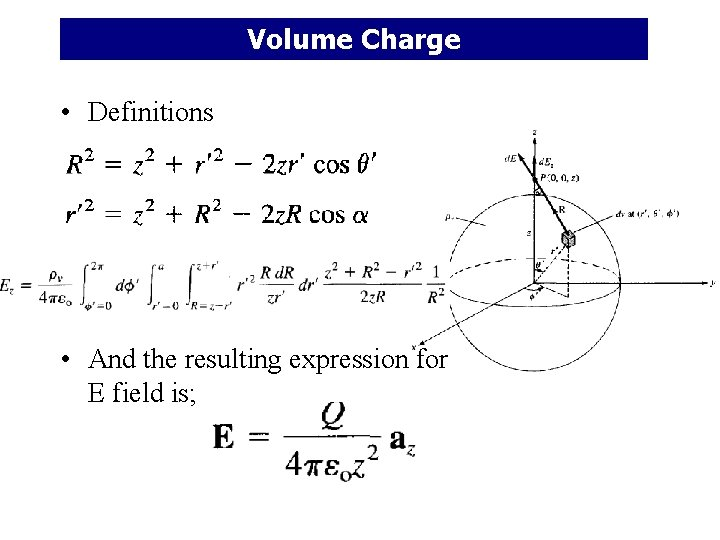Volume Charge • Definitions • And the resulting expression for E field is;Summarizing: Charge distributed along a line: Charge distributed over a surface: Charge distributed inside a volume: If the charge distribution is uniform, then , , and can be taken outside the integrals.The Electric Field Due to a Continuous Charge Distribution (worked examples)Example: A rod of length L has a uniform charge per unit length and a total charge Q. Calculate the electric field at a point P along the axis of the rod at a distance d from one end. y P x d L Let’s put the origin at P. The linear charge density and Q are related by Let’s assume Q is positive.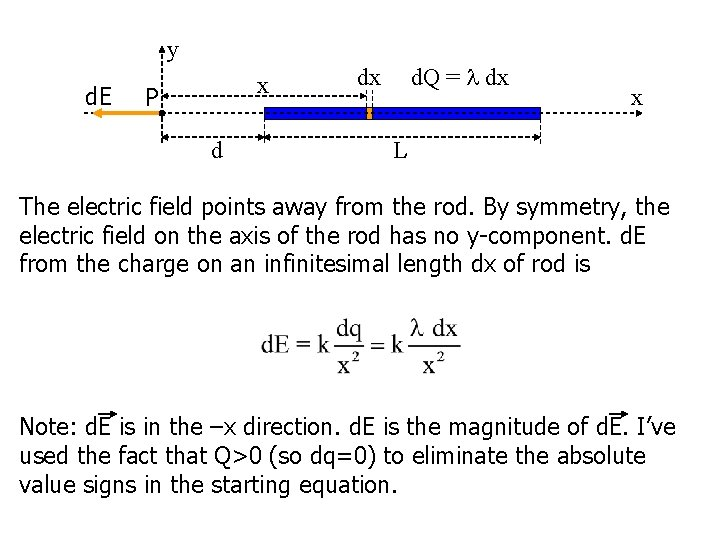y d. E x P d d. Q = dx dx x L The electric field points away from the rod. By symmetry, the electric field on the axis of the rod has no y-component. d. E from the charge on an infinitesimal length dx of rod is Note: d. E is in the –x direction. d. E is the magnitude of d. E. I’ve used the fact that Q>0 (so dq=0) to eliminate the absolute value signs in the starting equation.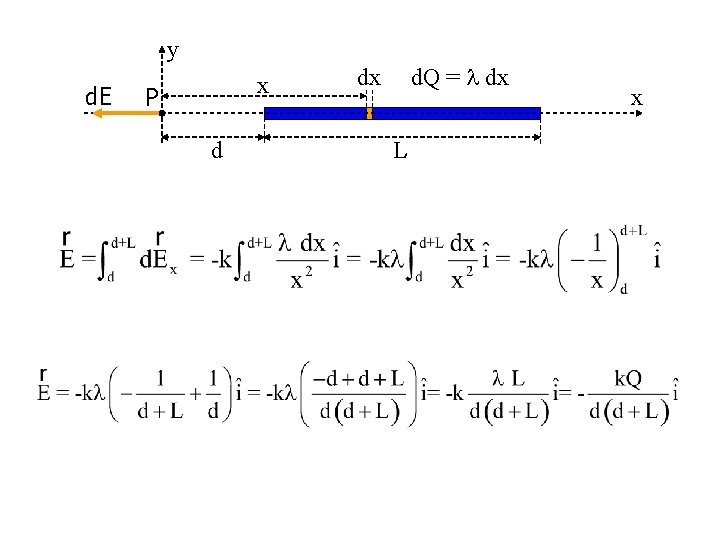y d. E x P d d. Q = dx dx L x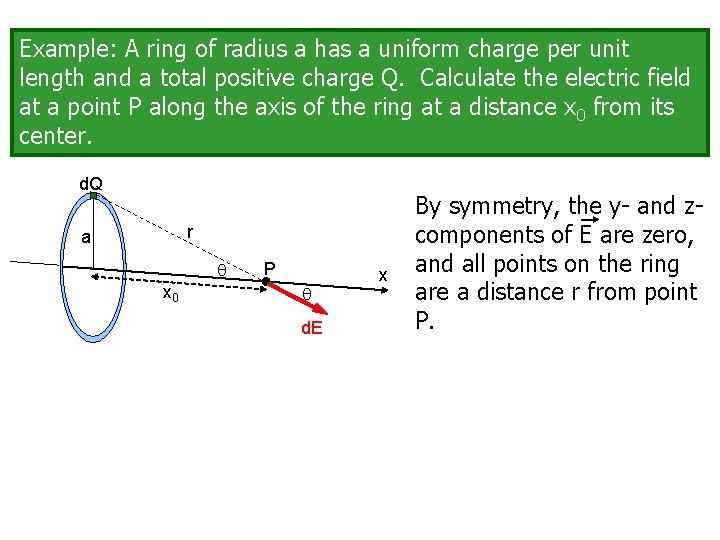Example: A ring of radius a has a uniform charge per unit length and a total positive charge Q. Calculate the electric field at a point P along the axis of the ring at a distance x 0 from its center. d. Q r a x 0 P d. E x By symmetry, the y- and zcomponents of E are zero, and all points on the ring are a distance r from point P.d. Q No absolute value signs because Q is positive. r a x 0 P x d. E For a given x 0, r is a constant for points on the ring. Or, in general, on the ring axis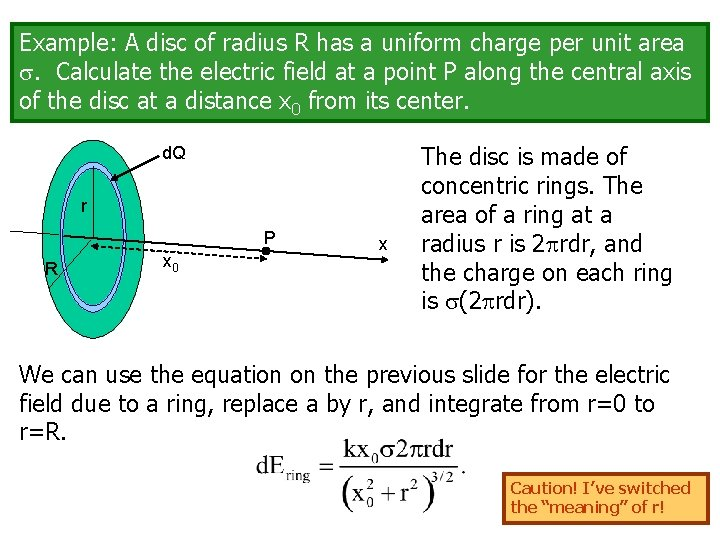Example: A disc of radius R has a uniform charge per unit area . Calculate the electric field at a point P along the central axis of the disc at a distance x 0 from its center. d. Q r P R x 0 x The disc is made of concentric rings. The area of a ring at a radius r is 2 rdr, and the charge on each ring is (2 rdr). We can use the equation on the previous slide for the electric field due to a ring, replace a by r, and integrate from r=0 to r=R. Caution! I’ve switched the “meaning” of r!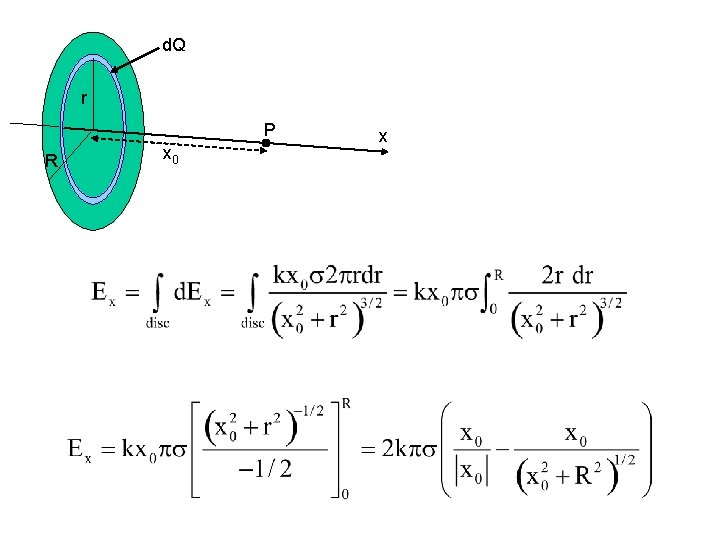d. Q r P R x 0 xExample: Calculate the electric field at a distance x 0 from an infinite plane sheet with a uniform charge density . Treat the infinite sheet as disc of infinite radius. Let R and use to get Interesting. . . does not depend on distance from the sheet. I’ve been Really Nice and put this on your starting equation sheet. You don’t have to derive it for your homework!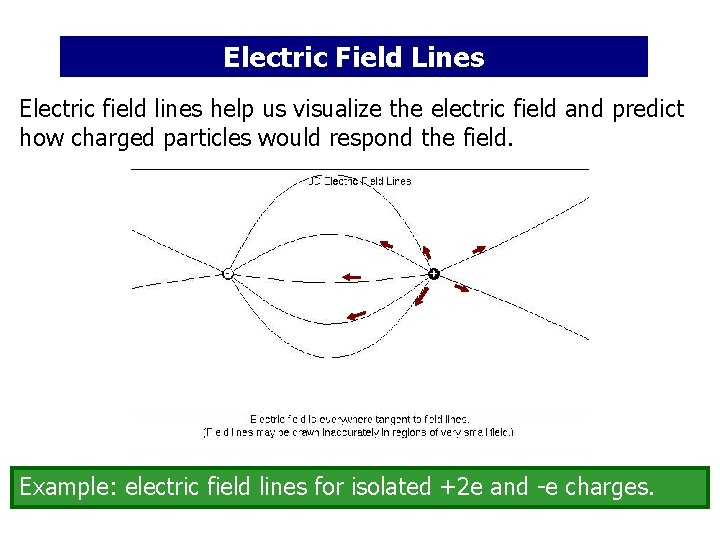Electric Field Lines Electric field lines help us visualize the electric field and predict how charged particles would respond the field. - + Example: electric field lines for isolated +2 e and -e charges.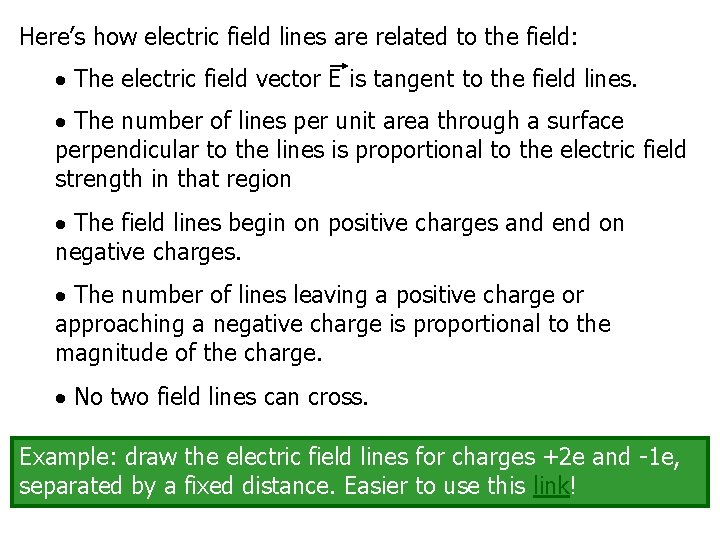Here’s how electric field lines are related to the field: The electric field vector E is tangent to the field lines. The number of lines per unit area through a surface perpendicular to the lines is proportional to the electric field strength in that region The field lines begin on positive charges and end on negative charges. The number of lines leaving a positive charge or approaching a negative charge is proportional to the magnitude of the charge. No two field lines can cross. Example: draw the electric field lines for charges +2 e and -1 e, separated by a fixed distance. Easier to use this link!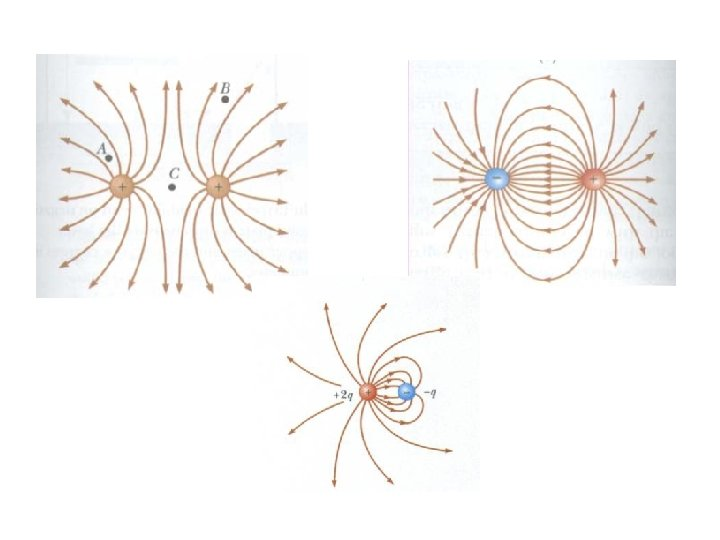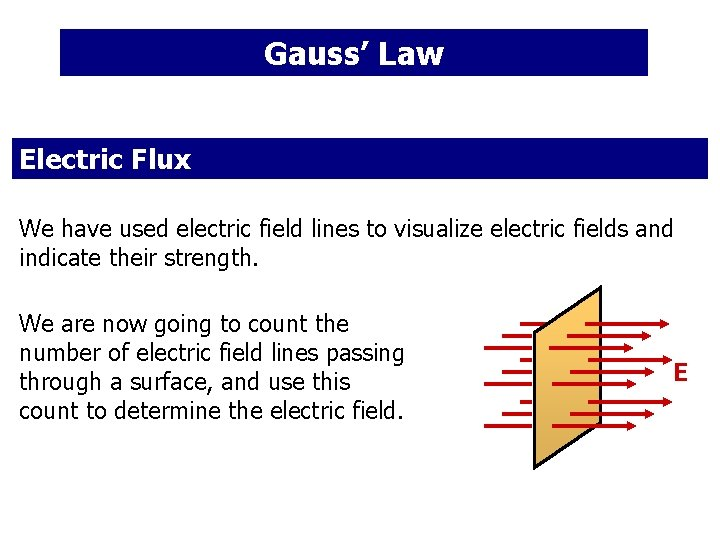Gauss’ Law Electric Flux We have used electric field lines to visualize electric fields and indicate their strength. We are now going to count the number of electric field lines passing through a surface, and use this count to determine the electric field. E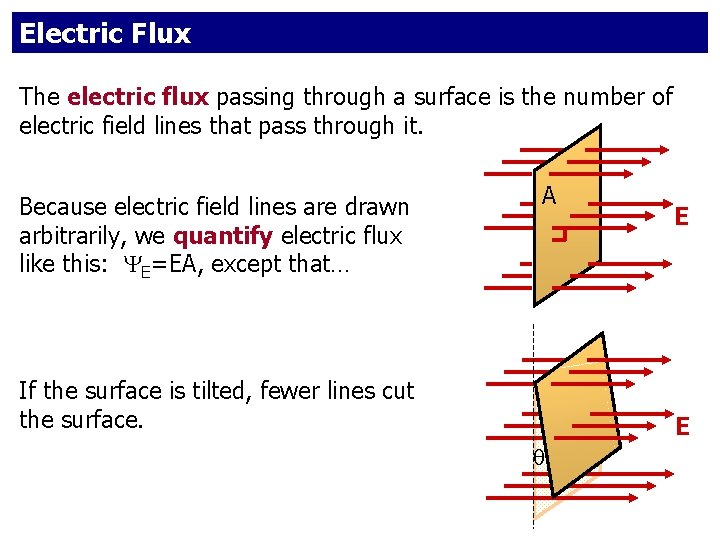Electric Flux The electric flux passing through a surface is the number of electric field lines that pass through it. Because electric field lines are drawn arbitrarily, we quantify electric flux like this: E=EA, except that… A If the surface is tilted, fewer lines cut the surface. E E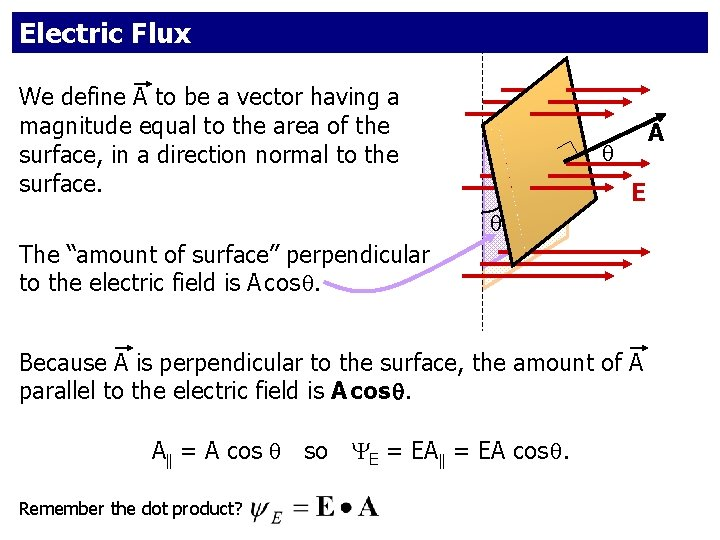Electric Flux We define A to be a vector having a magnitude equal to the area of the surface, in a direction normal to the surface. A E The “amount of surface” perpendicular to the electric field is A cos . Because A is perpendicular to the surface, the amount of A parallel to the electric field is A cos . A = A cos so E = EA cos . Remember the dot product?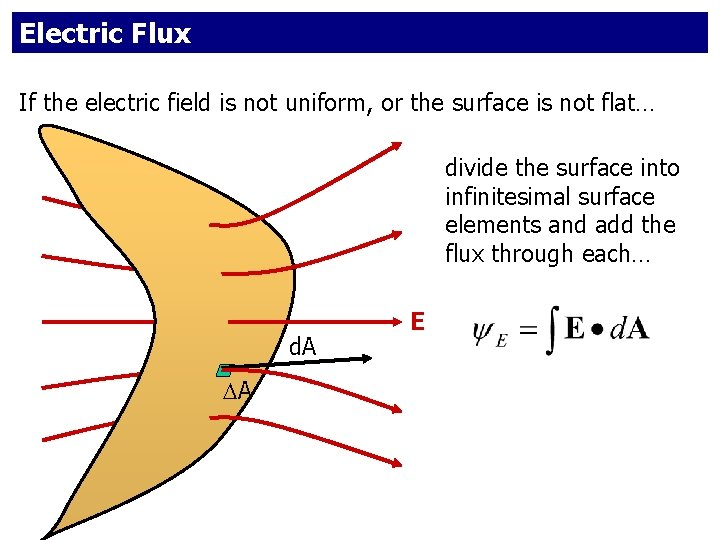Electric Flux If the electric field is not uniform, or the surface is not flat… divide the surface into infinitesimal surface elements and add the flux through each… d. A A E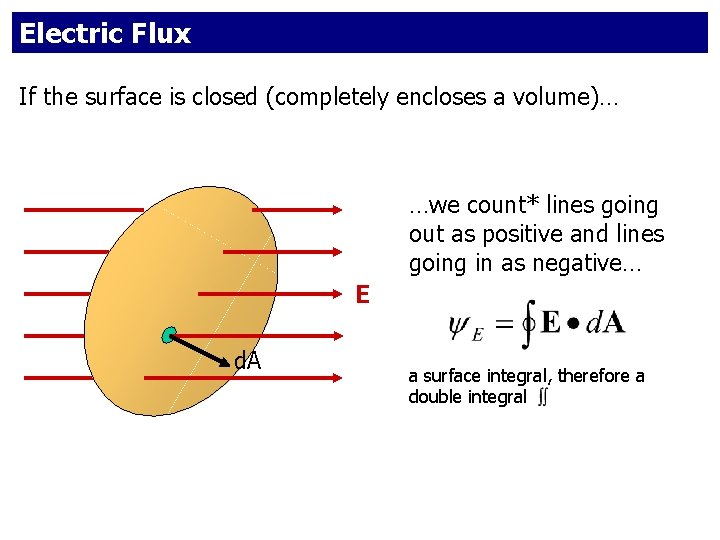Electric Flux If the surface is closed (completely encloses a volume)… …we count* lines going out as positive and lines going in as negative… E d. A a surface integral, therefore a double integralElectric Flux Use the simplest (easiest!) one that works. Flat surface, E A, E constant over surface. Easy! Flat surface, E not A, E constant over surface. Surface not flat, E not uniform. Avoid, if possible. Closed surface. Most general. Most complex. If the surface is closed, you may be able to “break it up” into simple segments and still use E=E·A for each segment.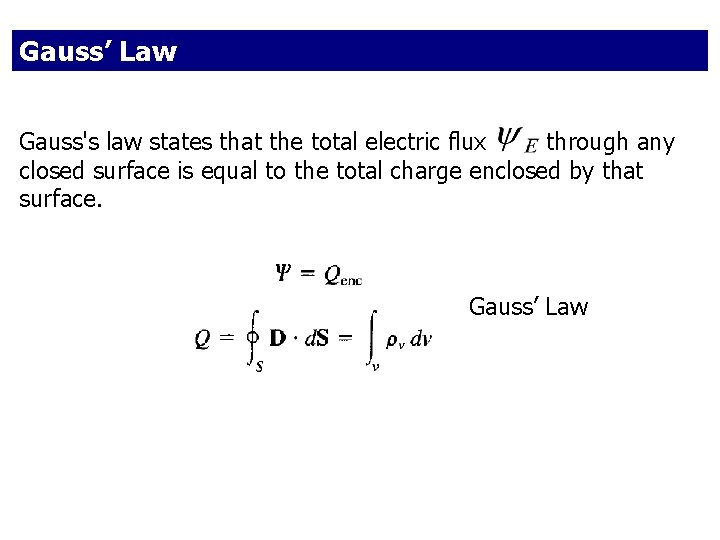Gauss’ Law Gauss's law states that the total electric flux through any closed surface is equal to the total charge enclosed by that surface. Gauss’ Law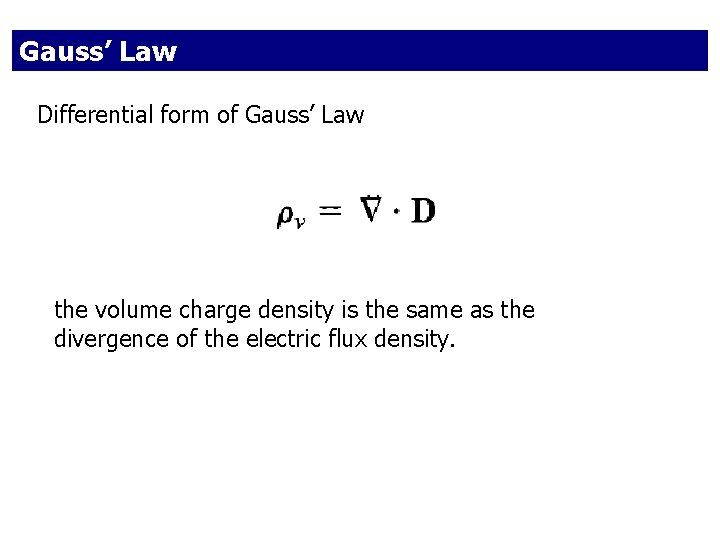Gauss’ Law Differential form of Gauss’ Law the volume charge density is the same as the divergence of the electric flux density.Gauss’ Law We will find that Gauss law gives a simple way to calculate electric fields for charge distributions that exhibit a high degree of symmetry…Point Charge Example: use Gauss’ Law to calculate the electric field from an isolated point positive charge q. To apply Gauss’ Law, we construct a “Gaussian Surface” enclosing the charge. The Gaussian surface should mimic the symmetry of the charge distribution. For this example, choose for our Gaussian surface a sphere of radius r, with the point charge at the center.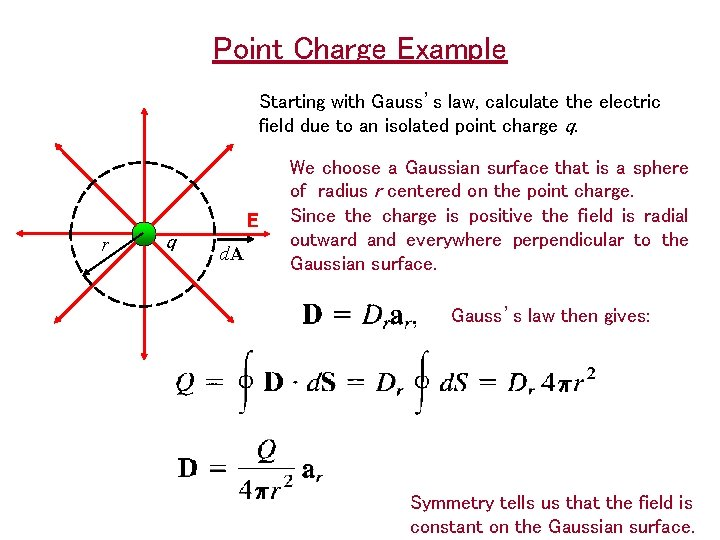Point Charge Example Starting with Gauss’s law, calculate the electric field due to an isolated point charge q. E r q d. A We choose a Gaussian surface that is a sphere of radius r centered on the point charge. Since the charge is positive the field is radial outward and everywhere perpendicular to the Gaussian surface. Gauss’s law then gives: Symmetry tells us that the field is constant on the Gaussian surface.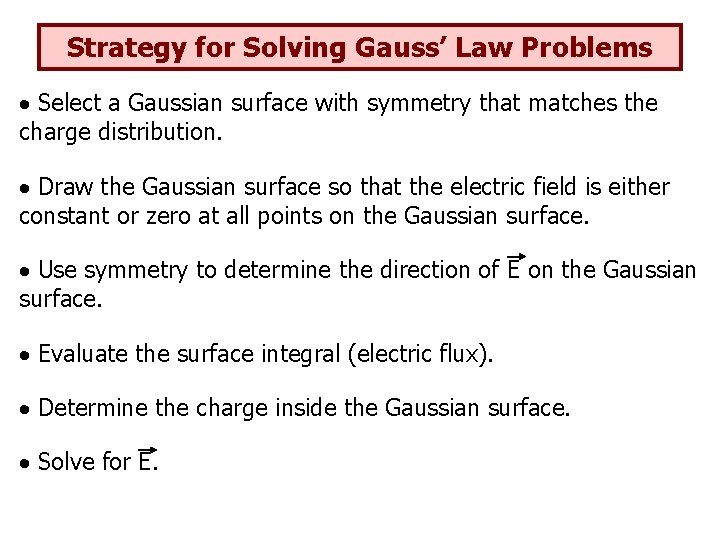Strategy for Solving Gauss’ Law Problems Select a Gaussian surface with symmetry that matches the charge distribution. Draw the Gaussian surface so that the electric field is either constant or zero at all points on the Gaussian surface. Use symmetry to determine the direction of E on the Gaussian surface. Evaluate the surface integral (electric flux). Determine the charge inside the Gaussian surface. Solve for E.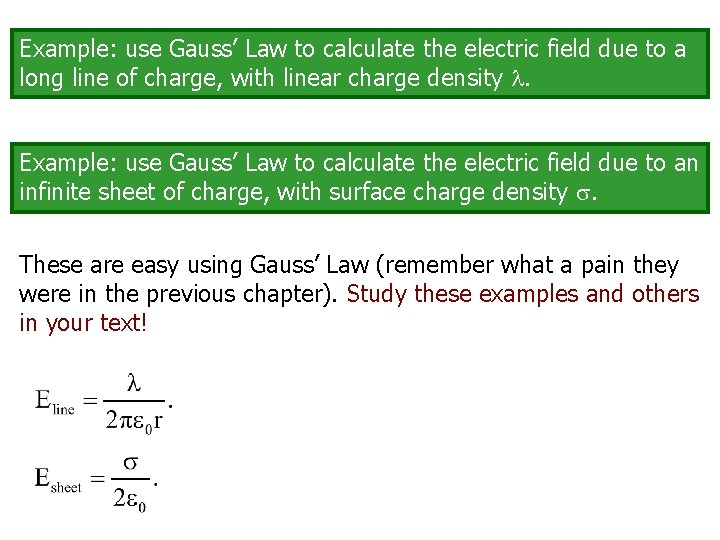Example: use Gauss’ Law to calculate the electric field due to a long line of charge, with linear charge density . Example: use Gauss’ Law to calculate the electric field due to an infinite sheet of charge, with surface charge density . These are easy using Gauss’ Law (remember what a pain they were in the previous chapter). Study these examples and others in your text!Worked Example 1 Compute the electric flux through a cylinder with an axis parallel to the electric field direction. E A The flux through the curved surface is zero since E is perpendicular to d. A there. For the ends, the surfaces are perpendicular to E, and E and A are parallel. Thus the flux through the left end (into the cylinder) is –EA, while the flux through right end (out of the cylinder) is +EA. Hence the net flux through the cylinder is zero.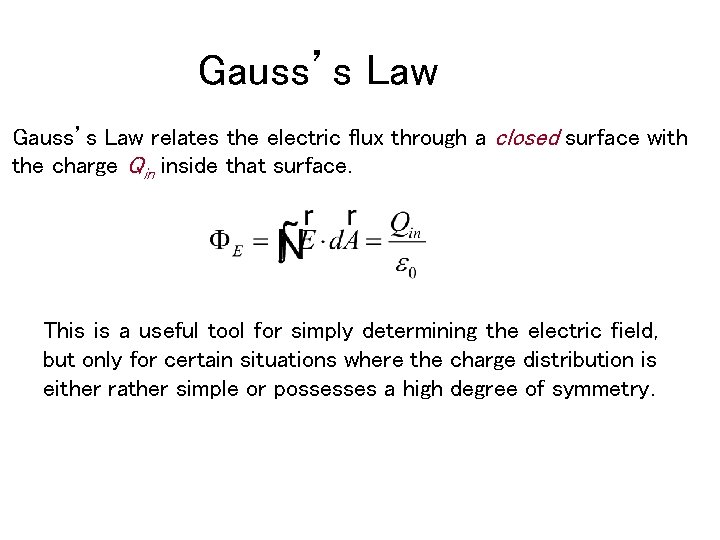Gauss’s Law relates the electric flux through a closed surface with the charge Qin inside that surface. This is a useful tool for simply determining the electric field, but only for certain situations where the charge distribution is either rather simple or possesses a high degree of symmetry.Worked Example 3 An insulating sphere of radius a has a uniform charge density ρ and a total positive charge Q. Calculate the electric field outside the sphere. Since the charge distribution is spherically symmetric we select a spherical Gaussian surface of radius r > a centered on the charged sphere. Since the charged sphere has a positive E r charge, the field will be directed radially outward. a d. A On the Gaussian sphere E is always parallel to d. A, and is constant. QWorked Example 3 cont’d r Find the electric field at a point inside the sphere. Now we select a spherical Gaussian surface with radius r < a. Again the symmetry of the charge distribution allows us to simply evaluate the left side of Gauss’s law just as before. a Q The charge inside the Gaussian sphere is no longer Q. If we call the Gaussian sphere volume V’ thenWorked Example 3 cont’d a Q E Let’s plot this: a rConductors in Electrostatic Equilibrium By electrostatic equilibrium we mean a situation where there is no net motion of charge within the conductor The electric field is zero everywhere inside the conductor Any net charge resides on the conductor’s surface The electric field just outside a charged conductor is perpendicular to the conductor’s surface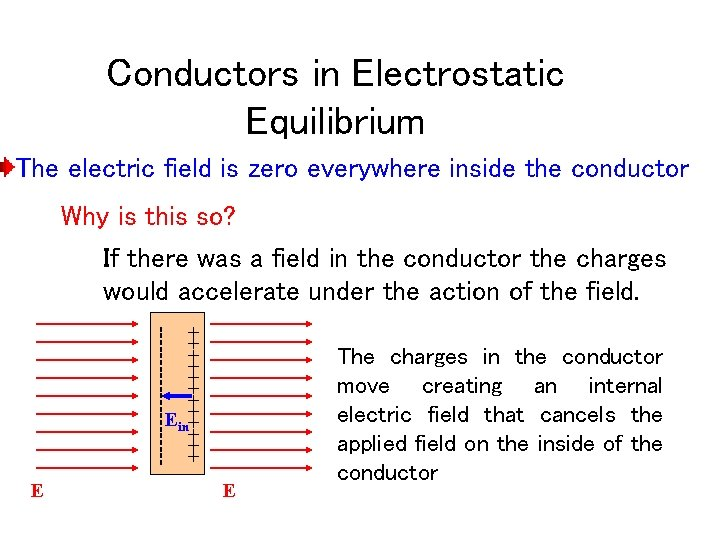Conductors in Electrostatic Equilibrium The electric field is zero everywhere inside the conductor Why is this so? If there was a field in the conductor the charges would accelerate under the action of the field. ++++++ ----------- Ein E E The charges in the conductor move creating an internal electric field that cancels the applied field on the inside of the conductorThe charge on the right is twice the magnitude of the charge on the left (and opposite in sign), so there are twice as many field lines, and they point towards the charge rather than away from it.Combinations of charges. Note that, while the lines are less dense where the field is weaker, the field is not necessarily zero where there are no lines. In fact, there is only one point within the figures below where the field is zero – can you find it?The electric field is always perpendicular to the surface of a conductor – if it weren’t, the charges would move along the surface.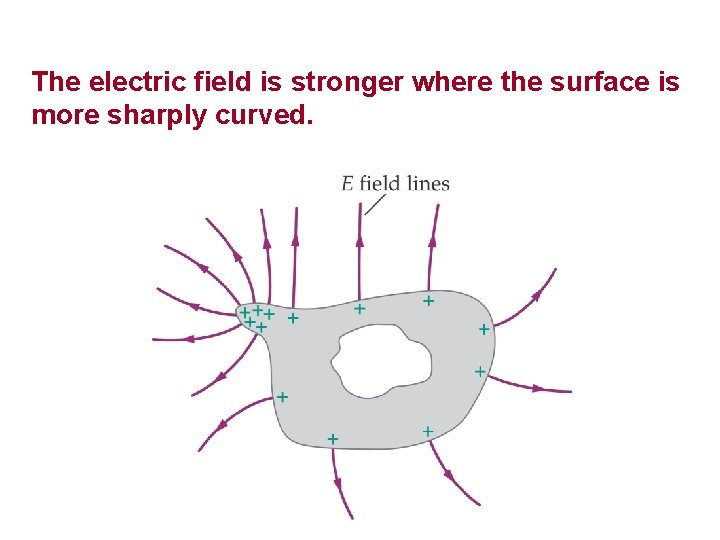The electric field is stronger where the surface is more sharply curved.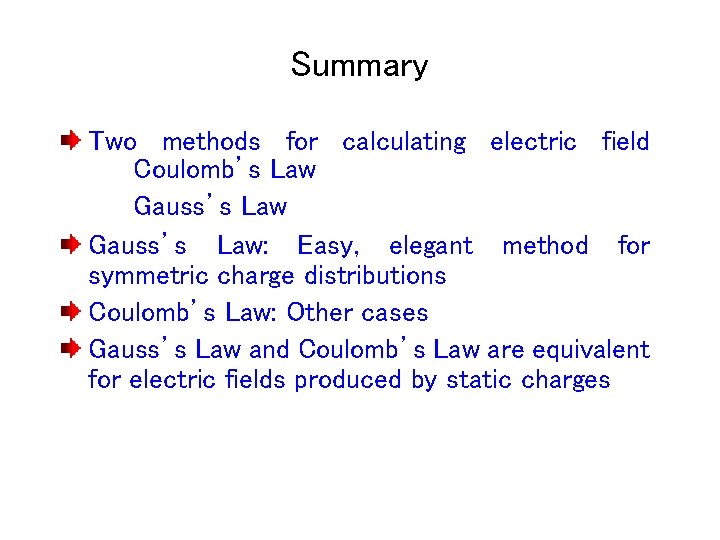Summary Two methods for calculating electric field Coulomb’s Law Gauss’s Law: Easy, elegant method for symmetric charge distributions Coulomb’s Law: Other cases Gauss’s Law and Coulomb’s Law are equivalent for electric fields produced by static chargesWorked Example 4 Any net charge on an isolated conductor must reside on its surface and the electric field just outside a charged conductor is perpendicular to its surface (and has magnitude σ/ε 0). Use Gauss’s law to show this. For an arbitrarily shaped conductor we can draw a Gaussian surface inside the conductor. Since we have shown that the electric field inside an isolated conductor is zero, the field at every point on the Gaussian surface must be zero. From Gauss’s law we then conclude that the net charge inside the Gaussian surface is zero. Since the surface can be made arbitrarily close to the surface of the conductor, any net charge must reside on the conductor’s surface.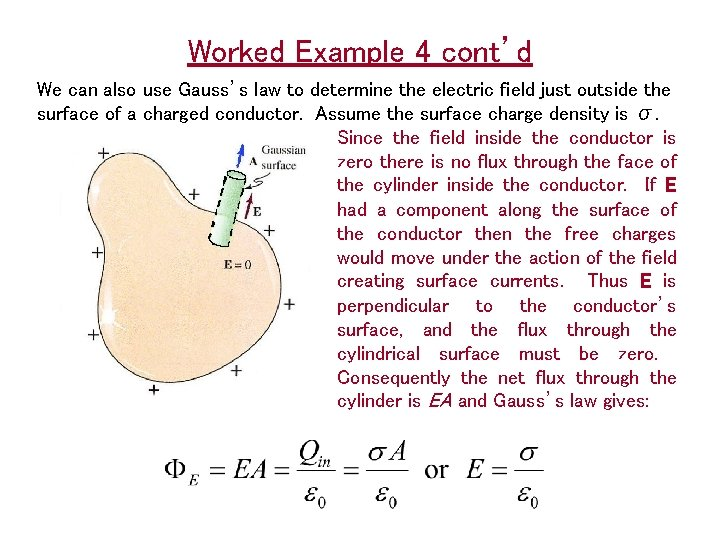Worked Example 4 cont’d We can also use Gauss’s law to determine the electric field just outside the surface of a charged conductor. Assume the surface charge density is σ. Since the field inside the conductor is zero there is no flux through the face of the cylinder inside the conductor. If E had a component along the surface of the conductor then the free charges would move under the action of the field creating surface currents. Thus E is perpendicular to the conductor’s surface, and the flux through the cylindrical surface must be zero. Consequently the net flux through the cylinder is EA and Gauss’s law gives:Worked Example 5 A conducting spherical shell of inner radius a and outer radius b with a net charge -Q is centered on point charge +2 Q. Use Gauss’s law to find the electric field everywhere, and to determine the charge distribution on the spherical shell. First find the field for 0 < r < a -Q This is the same as Ex. 2 and is the field due to a point charge with charge +2 Q. a +2 Q b Now find the field for a < r < b The field must be zero inside a conductor in equilibrium. Thus from Gauss’s law Qin is zero. There is a + 2 Q from the point charge so we must have Qa = 2 Q on the inner surface of the spherical shell. Since the net charge on the shell is -Q we can get the charge on the outer surface from Qnet = Qa + Qb. Qb= Qnet - Qa = -Q - (-2 Q) = + Q.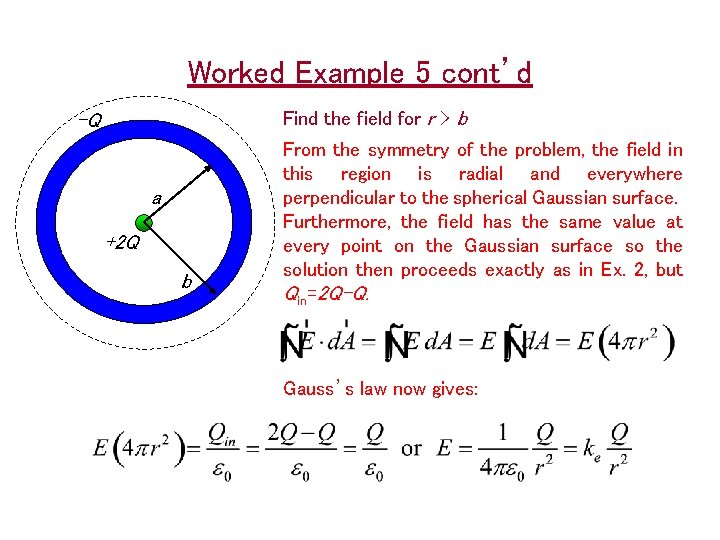Worked Example 5 cont’d Find the field for r > b -Q a +2 Q b From the symmetry of the problem, the field in this region is radial and everywhere perpendicular to the spherical Gaussian surface. Furthermore, the field has the same value at every point on the Gaussian surface so the solution then proceeds exactly as in Ex. 2, but Qin=2 Q-Q. Gauss’s law now gives: